—— 雪鸟对叶星，关于蓍叶烈焰焚河》，第5章

Snowbird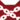至亲 (Q651)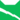雷族 (Q627)[临时][避难]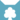天族 (Q632)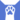反抗者 (Q4414)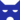影族 猫后影族 武士影族 猫后影族 武士影族 猫后天族 猫后影族 猫后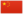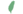雪鸟 Snowbird  雪鳥

Q3339：影族母猫，自《三力量》系列登场的角色

## 细节

### 外貌

• 她是一只身形轻盈、肌肉发达、皮毛光滑、绿色眼睛:猫物表的纯白色母猫，:猫物表其中一只脚掌已跛。:228

### 趣闻

• 她是目前拥有最多孩子的母猫，共三窝九只。:336:13章:30章

### 勘误

• 她最新的那窝孩子曾被错误地描述为两只。:14章

## 语录

—— 雪鸟，在细尾揭露鼠痕是叛徒后，群星之战，第279页

—— 雪鸟与黑星，在雪鸟要杀红柳时，《群星之战》，第280页

—— 雪鸟和蜂爪，在学徒们反叛时，雷影交加，第142-143页

—— 雪鸟对焦毛，关于洼光《雷影交加》，第323页

## 登场书目

• 预视力量 (Q152)[猫物闲角][首次登场🐱]
• 暗河汹涌 (Q153)[猫物闲角]
• 驱逐之战 (Q154)[配角]
• 天蚀遮月 (Q155)[配角]
• 暗夜长影 (Q156)[猫物闲角]
• 拂晓之光 (Q157)[猫物闲角]
• 第四学徒 (Q158)[猫物闲角]
• 战声渐近 (Q159)[配角]
• 暗夜密语 (Q160)[配角]
• 月光印记 (Q161)[猫物闲角]
• 武士归来 (Q162)[猫物闲角]
• 群星之战 (Q163)[配角]
• 学徒探索 (Q170)[对话提及]
• 雷影交加 (Q171)[配角]
• 天空破碎 (Q172)[配角]
• 极夜无光 (Q173)[配角]
• 烈焰焚河 (Q174)[配角]
• 风暴来袭 (Q175)[配角]
• 迷失群星 (Q3563)[配角]
• 静默冰融 (Q3579)[配角]
• 叠影重障 (Q3624)[配角]
• 暗由心生 (Q4183)[配角]
• 无星之地 (Q4385)[猫物闲角]
• 迷雾明光 (Q4621)[配角]
• 河翻浪涌 (Q4643)[配角]
• 黑莓星的风暴 (Q182)[对话提及]
• 虎心的阴影 (Q185)[配角]
• 鸦羽的拷问 (Q186)[猫物闲角]
• 松鼠飞的希冀 (Q3561)[配角]
• 灰条的誓言 (Q4057)[猫物闲角]
• 冬青叶的故事 (Q187)[猫物闲角]
• 雾星的征兆 (Q188)[猫物闲角]
• 鸽翅的沉默 (Q192)[猫物闲角]
• 褐皮的族群 (Q3566)[猫物闲角]
• 黛西的至亲 (Q3629)[猫物闲角]
• 斑毛的叛变 (Q4337)[猫物闲角]
• 族群决定 (Q4580)[配角]

• 消失的传统 （隐射）
• 至尊反派 （隐射）
• 创造反派 （隐射）
• 只是故事而已 （隐射）
• ## 脚注

1. 在书中，她并没有实际在断星、夜星和虎星的领导下出场过；但她是鼠痕的同窝，而他曾登场于《虎掌的愤怒》，也曾参与过偷取雷族幼崽的战斗；这表示她应该出生在森林，并参与过大迁徙。
2. 她在《驱逐之战》中是一名待产的猫后，但焦毛在《天蚀遮月》中还是学徒；凯特已证实莓心、苜蓿足和巴斯特出生于《天蚀遮月》，而焦毛正是他们的父亲。

## 参考文献

这篇文章基于CC BY-SA 3.0许可使用了猫武士维基（英语）Snowbird一文中的部分内容。
0.0
0人评价
岚掌 mistclaw
0

其实呢，她比香薇云更是超生队长能生，超生队队长为她最好（按生出来的只数算）（3+3+3=9只）不愧第一。

3年